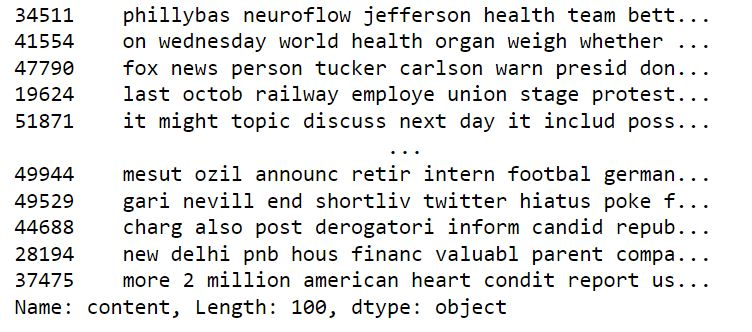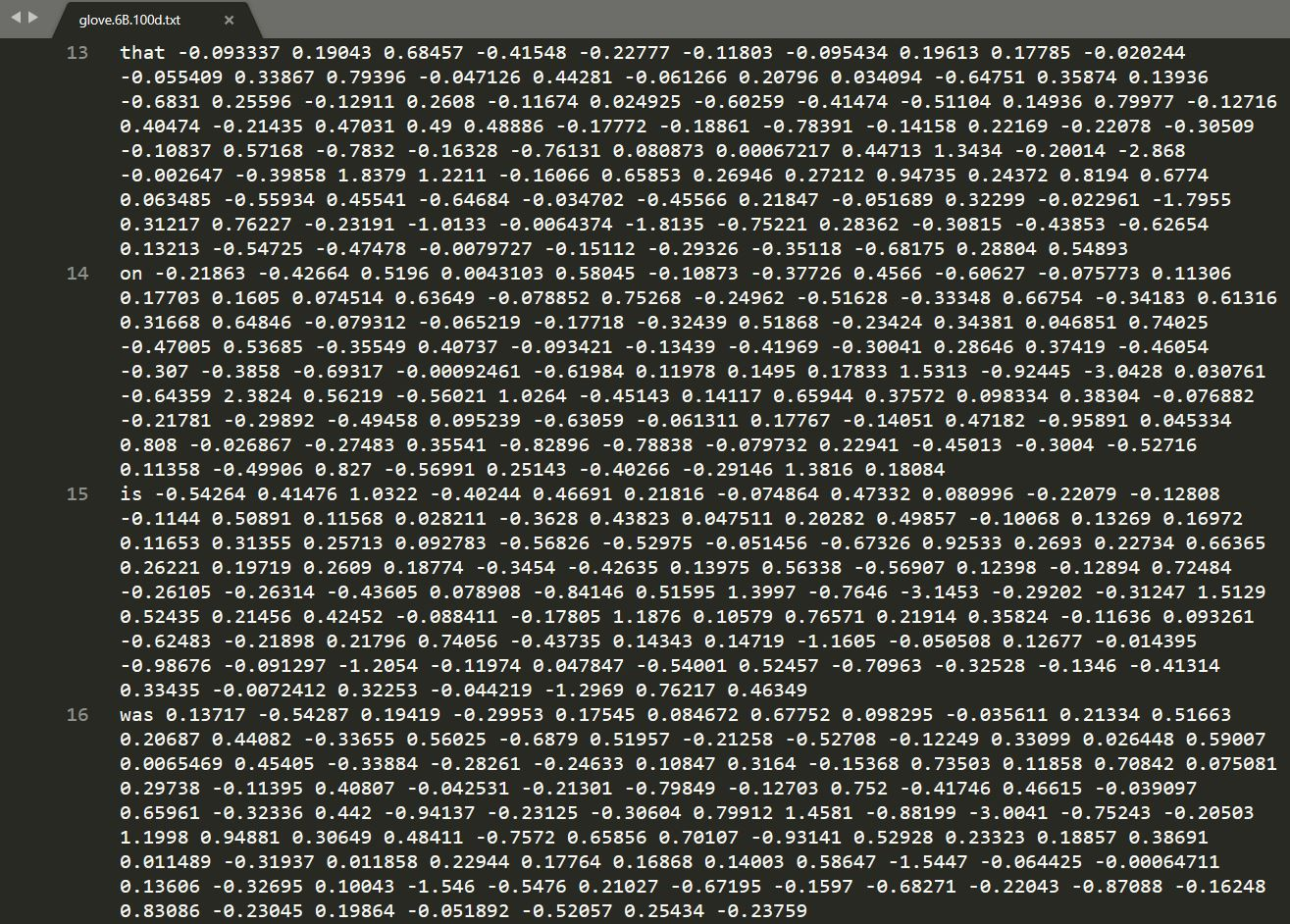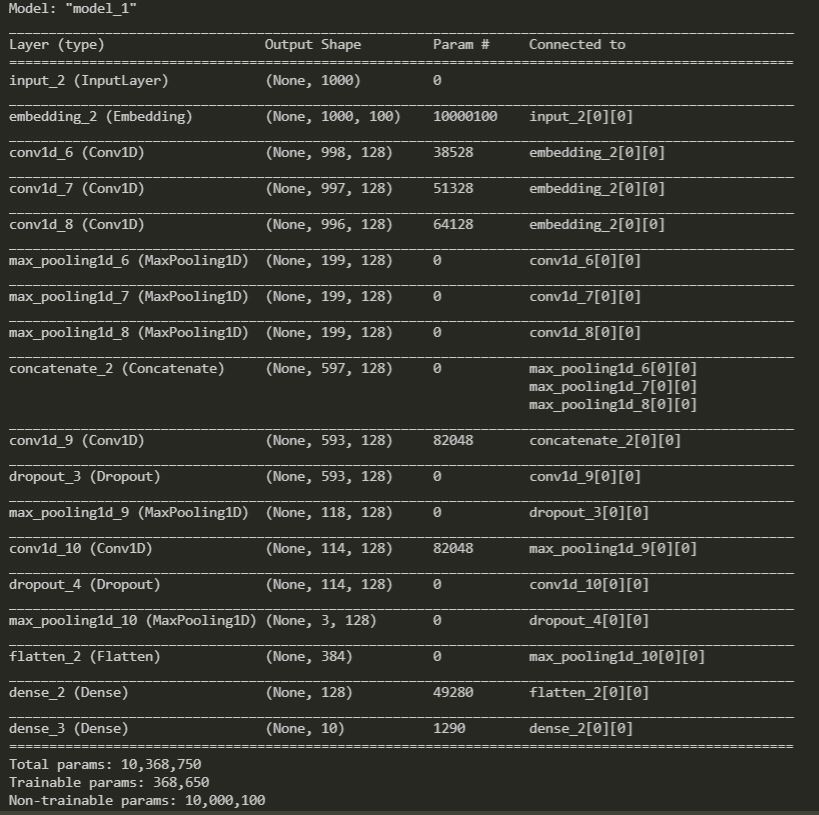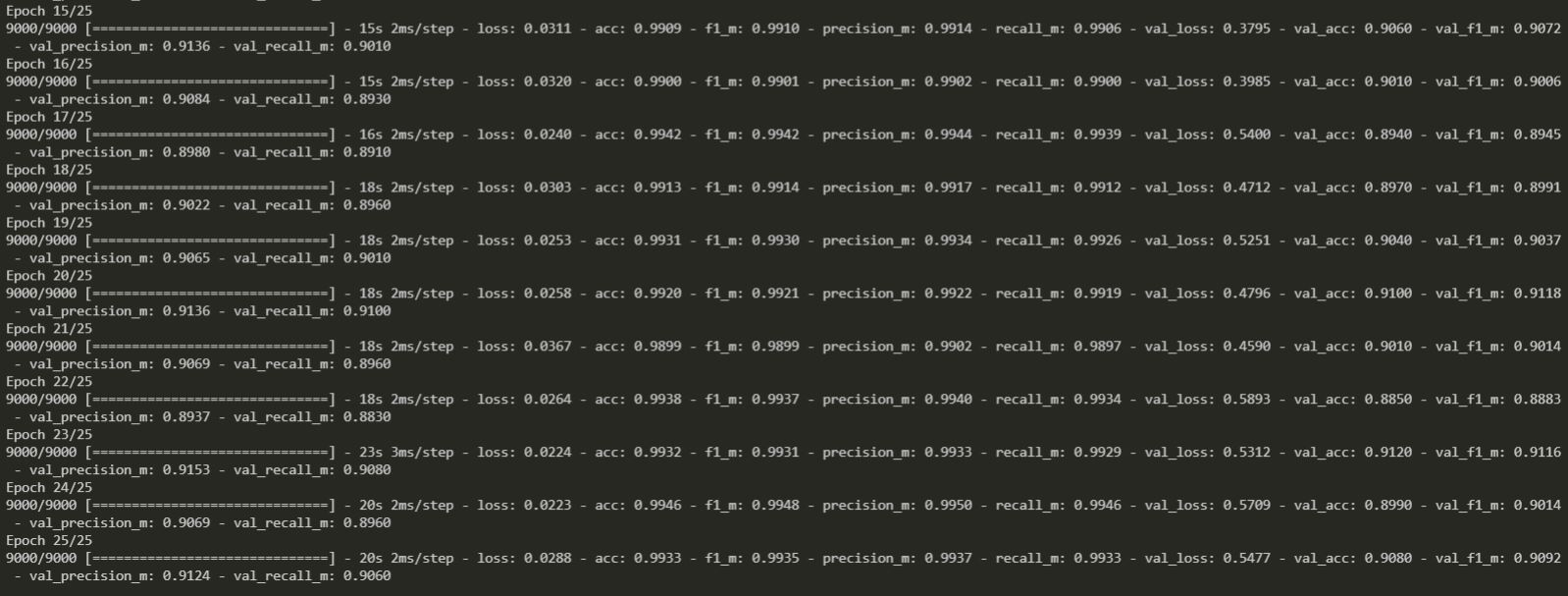Deep Learning(DL) is the subset of Machine Learning. It is a method of statistical learning that extracts features or attributes from raw data. DL uses a network of algorithms called artificial neural networks which imitates the function of the human neural networks present in the brain. DL takes the data into a network of layers(Input, Hidden & Output) to extract the features and to learn from the data.

In this blog, we will learn how to train a supervised text classification model using the DL python module called Keras and pre-trained GloVe word embeddings to transform the text data into a machine-understandable numerical representation. We will be using Convolutional Neural Networks(CNN) architecture to train the classification model.

The dataset and the category labels of the data are discussed in Text Classification using Machine Learning blog. Please refer to the blog and we will be using the same dataset here to train our CNN model to predict the classification of the given text.

Assume the dataset is referred to as the pandas dataframe called df in the code snippet.dataset

## Cleaning the dataset

The data cleaning part is also discussed in the blog.Cleaned Dataset

We have used stemming and stopwords removal on the dataset content. We can replace stemming with lemmatization and check out this blog about stemming vs lemmatization to know the differences. Skipping the cleaning part on the dataset for this DL blog, because stemming can give us meaningless words which don’t have the embedding glove vector.

As a part of data preparation, we are going to perform these operations on the dataset df

1. Lowering the content because the glove embedding vectors are generated for the lower case words.
2. Stripping and making sure the word starts with alphanumerics ie., removing special characters.
3. Dropping the null and empty columns from the df.
4. Dropping the duplicates from the df.

df = df[[‘content’, ‘label’]]
df = df.astype(‘str’).applymap(str.lower)
df = df.applymap(str.strip).replace(r”[^a-z0-9 ]+”, ”)
df = df.dropna()
df = df.drop_duplicates()

GloVe is an unsupervised learning algorithm for obtaining vector representations for words. Trained the models on Wiki, Twitter and common crawled data to have pre-trained word vectors with differences in size, tokens, and vocab size. For this blog, we will use the glove.6b.100d.txt pretrained glove word vector.Glove Embeddings

In the above image, we can see the words that, on, is, was is represented by vector coefficients.

embeddings_index = {}
for line in f:
values = line.split()
word = values
coefs = np.asarray(values[1:], dtype=’float32′)
embeddings_index[word] = coefs
f.close()
return embeddings_index

## Preparing the Embedding Matrix

MAX_NB_WORDS = 100000
def prepare_embedding_matrix(word_index):
“”” preparing embedding matrix with our data set “””
num_words = min(MAX_NB_WORDS, len(word_index))
embedding_matrix = np.zeros((num_words + 1, EMBEDDING_DIM))
for word, i in word_index.items():
if i >= MAX_NB_WORDS:
continue
embedding_vector = embeddings_index.get(word)
if embedding_vector is not None:
embedding_matrix[i] = embedding_vector
return embedding_matrix, num_words
MAX_NB_WORDS is the maximum number of words to consider as features for tokenizer
word_index is the tokenizer unique words list which extracted by fitting on our dataset content
The minimum of these two parameters is the num_words, which we keep as input_dim for the Keras Embedding layer.

## Preparing the dataset for the model to train

MAX_SEQUENCE_LENGTH = 1000
VALIDATION_SPLIT = 0.1
def vectorizing_data(df):
“”” vectorizing and splitting the data for training, testing, validating “””
label_s = df[‘label’].tolist()
l = list(set(label_s))
l.sort()
labels_index = dict([(j,i) for i, j in enumerate(l)])
labels = [labels_index[i] for i in label_s]
print(‘Found %s texts.’ % len(df[‘content’]))
print(‘labels_index — ‘, labels_index)
tokenizer = Tokenizer(num_words=MAX_NB_WORDS)
tokenizer.fit_on_texts(df[‘content’])
sequences = tokenizer.texts_to_sequences(df[‘content’])
word_index = tokenizer.word_index
print(‘Found %s unique tokens.’ % len(word_index))
labels = to_categorical(np.asarray(labels))
# randomizing and splitting the df into a training set, test set and a validation set
indices = np.arange(df.shape)
np.random.shuffle(indices)
df = df[indices]
labels = labels[indices]
num_validation_samples = int(VALIDATION_SPLIT * df.shape)
x_train = df[:-num_validation_samples]
y_train = labels[:-num_validation_samples]
x_val = df[-num_validation_samples:]
y_val = labels[-num_validation_samples:]
x_test = x_train[-num_validation_samples:]
y_test = y_train[-num_validation_samples:]
return x_train, y_train, x_test, y_test, x_val, y_val, word_index
Split the dataset for train, test, and validation purposes. Created a tokenizer and generated the word_index on the dataset content. Padded the sequences with the max length of MAX_SEQUENCE_LENGTH.

## Model construction

EMBEDDING_DIM = 100
def model_generation(embedding_matrix, num_words):
“”” model generation “””
embedding_layer = Embedding(num_words + 1,
EMBEDDING_DIM,
weights=[embedding_matrix],
input_length=MAX_SEQUENCE_LENGTH,
trainable=False)
convs = []
filter_sizes = [3,4,5]
sequence_input = Input(shape=(MAX_SEQUENCE_LENGTH,), dtype=’int32′)
embedded_sequences = embedding_layer(sequence_input)
for fsz in filter_sizes:
l_conv = Conv1D(filters=128, kernel_size=fsz, activation=’relu’)(embedded_sequences)
l_pool = MaxPooling1D(5)(l_conv)
convs.append(l_pool)
l_merge = Concatenate(axis=1)(convs)
l_cov1= Conv1D(filters=128, kernel_size=5, activation=’relu’)(l_merge)
l_cov1 = Dropout(0.2)(l_cov1)
l_pool1 = MaxPooling1D(5)(l_cov1)
l_cov2 = Conv1D(filters=128, kernel_size=5, activation=’relu’)(l_pool1)
l_cov2 = Dropout(0.2)(l_cov2)
l_pool2 = MaxPooling1D(30)(l_cov2)
l_flat = Flatten()(l_pool2)
l_dense = Dense(128, activation=’relu’)(l_flat)
preds = Dense(label_count, activation=’softmax’)(l_dense)
model = Model(sequence_input, preds)
return model
The model summary looks like thisModel Summary

The model is represented by the embedding layer followed by convolutional layers, pooling layers, and dropout layers. The final layer is the dense layer with the output size of labels/category count.

Dropout is dropping off the neurons to prevent an over-fitting problem in neural networks. It is an approach to regularization in neural networks which helps to reduce interdependent learning amongst the neurons. In Machine Learning we use regularization to prevent an over-fitting problem by adding a penalty to the loss function.

Batch normalization is another method to regularize a convolutional network.

## f1-score, precision, and recall

def recall_m(y_true, y_pred):
true_positives = K.sum(K.round(K.clip(y_true * y_pred, 0, 1)))
possible_positives = K.sum(K.round(K.clip(y_true, 0, 1)))
recall = true_positives / (possible_positives + K.epsilon())
return recall
def precision_m(y_true, y_pred):
true_positives = K.sum(K.round(K.clip(y_true * y_pred, 0, 1)))
predicted_positives = K.sum(K.round(K.clip(y_pred, 0, 1)))
precision = true_positives / (predicted_positives + K.epsilon())
return precision
def f1_m(y_true, y_pred):
precision = precision_m(y_true, y_pred)
recall = recall_m(y_true, y_pred)
return 2*((precision*recall)/(precision+recall+K.epsilon()))
These parameters evaluate the trained model. f1-score is the harmonic mean of precision and recall.
Precision is calculated as the number of true positives divided by the total number of true positives and false positives.
Recall is calculated as the number of true positives divided by the total number of true positives and false negatives.

## Model training and evaluation

def training_evaluating_model(model, x_train, y_train, x_test, y_test, x_val, y_val):
“”” training the model with the train and validation data
and evaluating the model with the test data “””
model.compile(loss=’categorical_crossentropy’,
optimizer=’rmsprop’,
metrics=[‘acc’, f1_m, precision_m, recall_m])
# Displays the network structure
model.summary()
# fitting the model
model.fit(x_train, y_train, validation_data=(x_val, y_val), epochs=epochs, batch_size=batch_size)
“””
model.save_weights(home_path + ‘model_trained’) # Saving the model
“””
# evaluating the model
loss, accuracy, f1_score, precision, recall = model.evaluate(x_test, y_test, verbose=0)
return loss, accuracy, f1_score, precision, recallModel Evaluation

The model training accuracy is around 99.33% and validation accuracy is around 90.8%. Validation loss is more compared to training loss which resulted in the reduction of validation accuracy.  We have trained on dataset sample of 10000 rows only, if we have trained on complete dataset and increase in the number of epochs, results would have been much better.

The complete code discussed above can be found here.